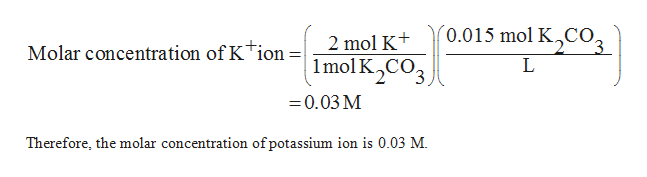# What is the molar concentration of K+ ions in a 0.015 M solution of potassium carbonate?

Question
308 views
• What is the molar concentration of K+ ions in a 0.015 M solution of potassium carbonate?
check_circle

Step 1

The molar concentration of the substance can be described as the ratio of the moles of the substance to the volume of the solution in the liters.

Step 2

The equation for the dissociation of potassium carbonate is shown below:

Step 3

In the above equation, one mole potassium carbonate is dissociated to form two moles of potassium ion and ...help_outlineImage Transcriptionclose´0.015 mol K,CO3 2 mol K+ Molar concentration of Ktion = 1mol K,CO3 = 0.03 M Therefore, the molar concentration of potassium ion is 0.03 M. fullscreen

### Want to see the full answer?

See Solution

#### Want to see this answer and more?

Solutions are written by subject experts who are available 24/7. Questions are typically answered within 1 hour.*

See Solution
*Response times may vary by subject and question.
Tagged in

### Chemistry